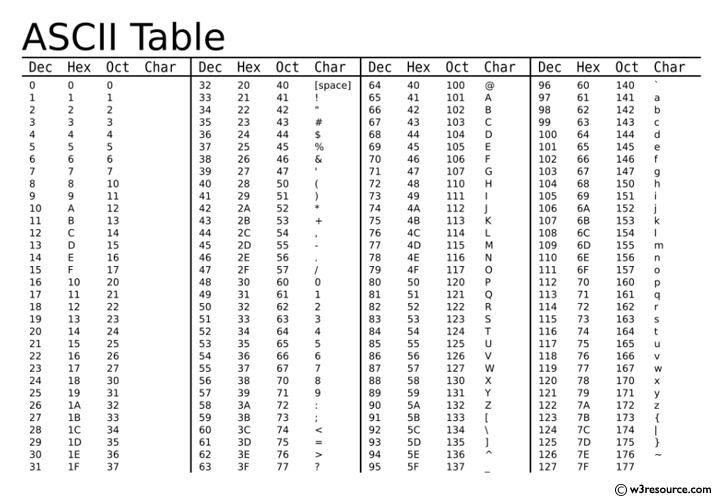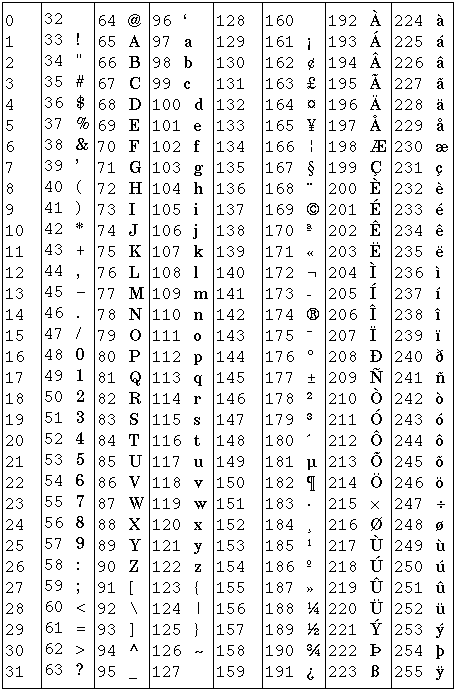Related Tags

python
community creator

# What are ord() and chr() functions in Python?

B. Sree Vaishnavi

## ord() function

The ord() function is a built-in function in Python that converts a specified character into an integer

### Syntax:

ord(a)


Example: A string of length 1 returns an integer representing the Unicode code point of the character when an argument is a Unicode object or the byte’s value when the argument is an 8-bit string. So, if we take ord(‘a’) it returns the integer 97, and ord(‘€’) (Euro sign) returns 8364.

Note: If the length of a string is more than one, a TypeError will be raised.

print(ord('A'))
print(ord('a'))
print(ord('&'))

### ASCII table

Below is an ASCII table, use this for reference.## chr() function

The chr() function is a built-in Python function that converts a specified integer value into a character.

### Syntax:

String chr(n)


The valid range for n is 0 to 1,114,111.

The chr() function returns a string that represents a character whose Unicode code point value is n.

Note: If an integer is passed outside the range, the method returns a ValueError.

### Example

print(chr(65))
print(chr(36))
print(chr(97))

### Unicode character

Below is a Unicode character set table, use this for reference.RELATED TAGS

python
community creator

CONTRIBUTOR

B. Sree Vaishnavi
RELATED COURSES

View all Courses

Keep Exploring

Learn in-demand tech skills in half the time